# Test Prep Plan - Take a practice test

Take this practice test to check your existing knowledge of the course material. We'll review your answers and create a Test Prep Plan for you based on your results.
How Test Prep Plans work
1
2Based on your results, we'll create a customized Test Prep Plan just for you!
3Study smarter
Study more effectively: skip concepts you already know and focus on what you still need to learn.

# Functions - Basics for Precalculus: Homework Help Chapter Exam

Exam Instructions:

Choose your answers to the questions and click 'Next' to see the next set of questions. You can skip questions if you would like and come back to them later with the yellow "Go To First Skipped Question" button. When you have completed the practice exam, a green submit button will appear. Click it to see your results. Good luck!

### Page 1

#### Question 2 2. Choose the answer that best describes the function of this graph.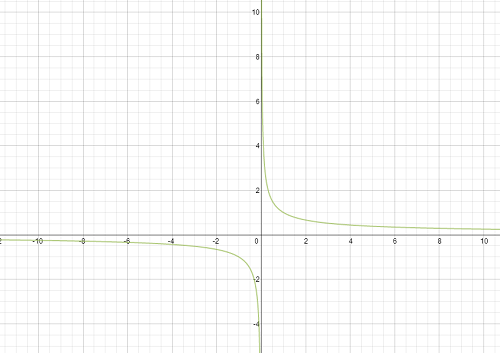#### Question 3 3. What is the domain of the function below?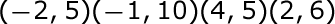#### Question 4 4. Choose the answer that best describes the function of this graph.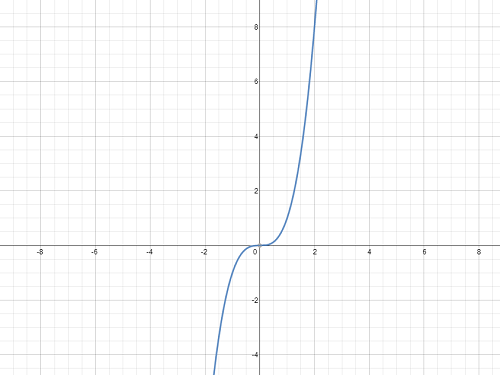### Page 3

#### Question 14 14. Choose the answer that best describes the function of this graph. Look at the graph that is shown below. Which answer choice best describes the function of this graph?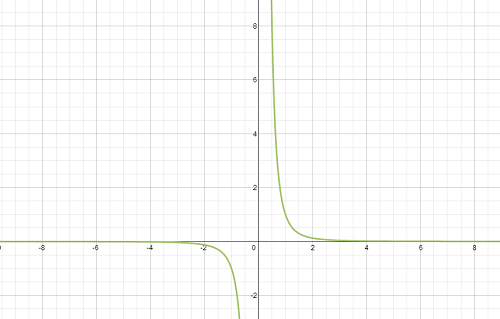### Page 4

#### Question 16 16. Which equation matches the following graph?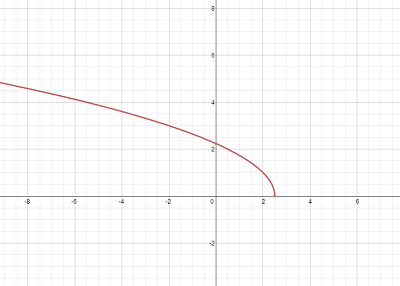#### Question 17 17. Which kind of transformation would change the purple function below into the yellow one?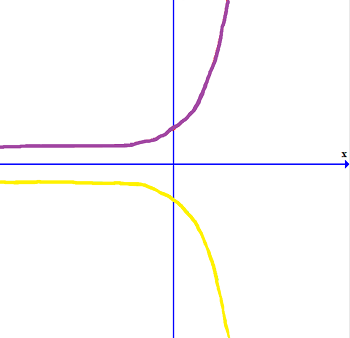#### Question 18 18. Choose the answer that best describes the function of this graph.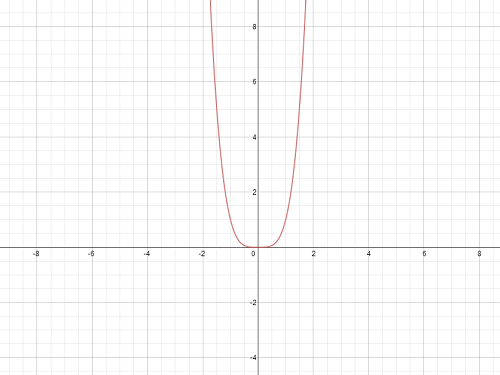#### Question 19 19. Given the function below, evaluate g(7).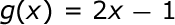### Page 5

#### Question 22 22. Which equation matches the following graph?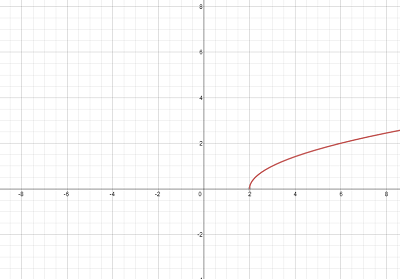#### Question 23 23. What is the range of the function represented by the graph below?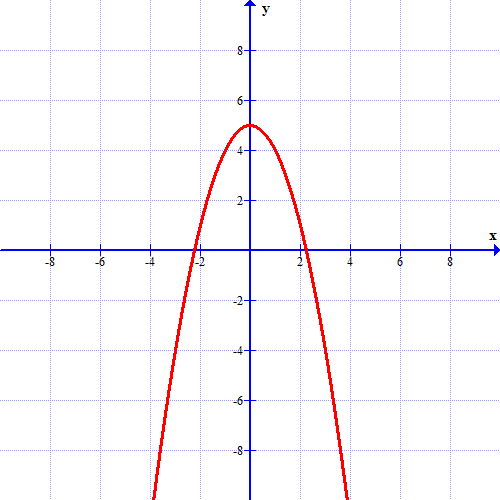#### Question 24 24. Choose the answer that best describes the function of this graph. Select the best answer choice that describes the function of this graph.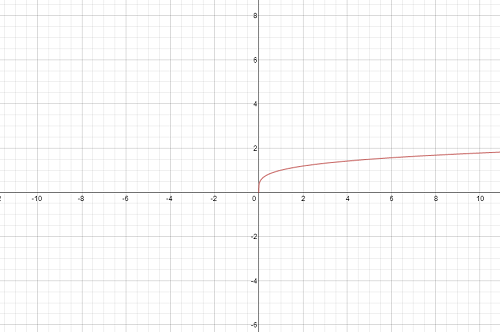#### Question 25 25. Which equation matches the following graph?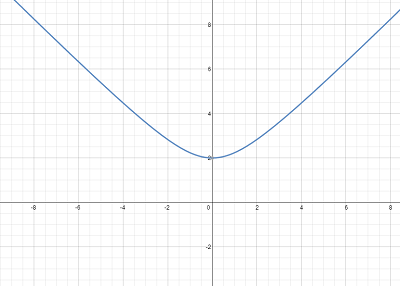### Page 6

#### Question 27 27. In the figure shown below, the graph of the yellow function is a translation of the graph of the blue function. If the expression for the blue function is y(x) = b(x), which of the following is the equation of the yellow function?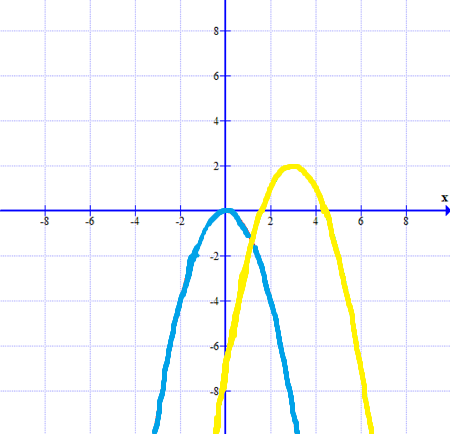#### Question 28 28. If the graph of f(x) is shown below, which choice represents the graph of f(x + 1) + 2?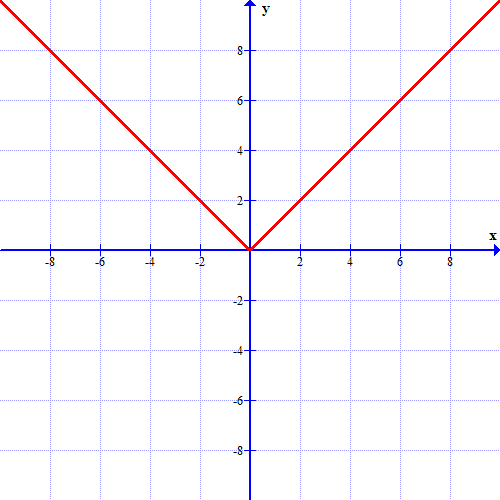#### Functions - Basics for Precalculus: Homework Help Chapter Exam Instructions

Choose your answers to the questions and click 'Next' to see the next set of questions. You can skip questions if you would like and come back to them later with the yellow "Go To First Skipped Question" button. When you have completed the practice exam, a green submit button will appear. Click it to see your results. Good luck!

Support